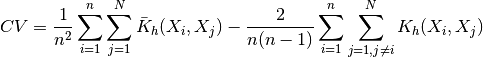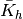# statsmodels.nonparametric.kernel_density.KDEMultivariate.imse¶

KDEMultivariate.imse(bw)[source]

Returns the Integrated Mean Square Error for the unconditional KDE.

Parameters: bw: array_like The bandwidth parameter(s). CV: float The cross-validation objective function.

Notes

See p. 27 in [R6] for details on how to handle the multivariate estimation with mixed data types see p.6 in [R7].

The formula for the cross-validation objective function is:Whereis the multivariate product convolution kernel (consult [R7] for mixed data types).

References

 [R6] (1, 2) Racine, J., Li, Q. Nonparametric econometrics: theory and practice. Princeton University Press. (2007)
 [R7] (1, 2, 3) Racine, J., Li, Q. “Nonparametric Estimation of Distributions with Categorical and Continuous Data.” Working Paper. (2000)